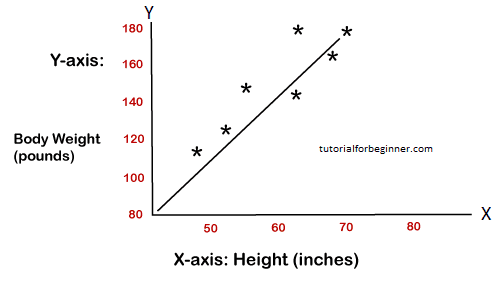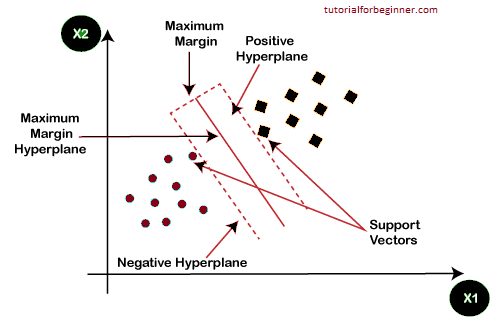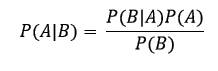# Machine Learning Algorithms

In this page, We will learn about Machine Learning Algorithms, Types of Machine Learning Algorithms, Supervised Learning Algorithm, Unsupervised Learning Algorithm, Reinforcement Learnin, Linear Regression, Logistic Regression, Decision Tree Algorithm, Support Vector Machine Algorithm, Naïve Bayes Algorithm, K-Nearest Neighbour (KNN), K-Means Clustering, Random Forest Algorithm, Apriori Algorithm, and Principle Component Analysis.

Machine Learning algorithms are systems that can self-learn hidden patterns in data, forecast output, and enhance performance based on previous experiences. In machine learning, multiple algorithms can be employed for different tasks, such as basic linear regression for prediction problems like stock market forecasting and the KNN algorithm for categorization challenges.

We'll look at some of the most popular and widely used machine learning algorithms, as well as their use cases and categories, in this topic.

## Types of Machine Learning Algorithms

Machine Learning Algorithm can be broadly divided into three types:

The below figure illustrates the different ML algorithm, along with the categories:## 1. Supervised Learning Algorithm

Supervised learning is a sort of machine learning in which the machine learns with the help of another person. The labeled dataset is used to train the supervised learning models. After the model has been trained and processed, it is tested by supplying a sample of test data to see if it correctly predicts the output.

In supervised learning, the goal is to map input data to output data. Supervised learning is based on monitoring, and it is similar to when a student learns under the supervision of a teacher. Spam filtering, price detection is one example of supervised learning.

The challenge of supervised learning can be further separated into two categories:

• Classification
• Regression

Examples of some popular supervised learning algorithms are Simple Linear regression, Decision Tree, Logistic Regression, KNN algorithm, etc. Read more..

## 2) Unsupervised Learning Algorithm

Unsupervised learning is a sort of machine learning in which the system learns from data without the requirement for external supervision. Unsupervised models can be trained using an unlabeled dataset that is neither classified nor categorized, and the algorithm must act on it without supervision. The model in unsupervised learning doesn't have a specified output and instead tries to extract meaningful information from a large amount of input. These are used to solve the challenges of association and clustering. As a result, it can be divided into two types:

• Classification
• Regression

Examples of some Unsupervised learning algorithms are K-means Clustering, Apriori Algorithm, Eclat, etc. Read more..

## 3) Reinforcement Learning

Reinforcement learning is a type of learning in which an agent interacts with its environment by producing actions and learns from the feedback it receives. The feedback is supplied to the agent in the form of rewards, such as a positive reward for each successful activity and a negative reward for each bad action. The agent does not receive any oversight. Reinforcement learning employs the Q-Learning algorithm.

List of Popular Machine Learning Algorithm

## 1. Linear Regression

For predictive analysis, linear regression is one of the most common and straightforward machine learning methods. Predictive analysis is used to describe something that can be predicted, and linear regression is used to predict continuous numbers like salary, age, and so on.

It depicts the dependent and independent variables' linear relationship, as well as how the dependent variable(y) varies when the independent variable changes (x).

It seeks to find the best fit line between the dependent and independent variables, which is called the regression line.

The regression line's equation is as follows:

### y = a0 + a*x+ b

Here, y= dependent variable
x= independent variable
a0 = Intercept of line.

The two types of linear regression are as follows:

• Simple Linear Regression: A single independent variable is used to predict the value of the dependent variable in simple linear regression.
• Multiple Linear Regression: Multiple independent variables are utilized to predict the value of the dependent variable in multiple linear regression.

The linear regression for weight prediction based on height is depicted in the picture below:## 2. Logistic Regression

The supervised learning process of logistic regression is used to predict categorical variables or discrete values. It can be used in machine learning for classification issues, and the result of the logistic regression algorithm can be Yes or No, 0 or 1, Red or Blue, and so on.

Logistic regression is comparable to linear regression in that it is used to solve the classification problem and predict discrete values, whereas linear regression is used to solve the regression problem and predict continuous values.

It produces an S-shaped curve between 0 and 1 instead of fitting the best fit line. The S-shaped curve is also referred to as a logistic function that employs the threshold notion. Any value greater than or equal to the threshold will tend to 1; any value less than or equal to the threshold will gravitate to 0.

## 3. Decision Tree Algorithm

A decision tree is a supervised learning technique that may be used to tackle classification and regression problems. It is capable of working with both categorical and continuous variables. It depicts a tree-like structure with nodes and branches, beginning with the root node and expanding on subsequent branches until reaching the leaf node. The internal node represents the dataset's features, while the branches reflect the decision rules and the leaf nodes indicate the problem's conclusion.

Real-world uses of decision tree algorithms include identifying malignant and non-cancerous cells, making car-buying recommendations, and so on.

## 4. Support Vector Machine Algorithm

A support vector machine, or SVM, is a supervised learning technique that can be used to solve issues like classification and regression. It is, however, mostly worked to solve categorization difficulties. The purpose of SVM is to generate a decision boundary or hyperplane that can divide datasets into multiple classes.

Support vectors are the data points that assist define the hyperplane, hence the algorithm is called support vector machine.

Face detection, picture categorization, drug discovery, and more real-world applications of SVM can be found. Consider the diagram below:As we can see in the above diagram, the hyperplane has classified datasets into two different classes.

## 5. Naïve Bayes Algorithm:

The Nave Bayes classifier is a supervised learning algorithm that makes predictions based on the object's likelihood. The algorithm is called Nave Bayes because it is based on the Bayes theorem and follows the naive assumption that variables are independent of one another.

The Bayes theorem is based on conditional probability, which refers to the likelihood that event(A) will occur if event(B) has previously occurred. The Bayes theorem's equation is as follows:The Nave Bayes classifier is one of the most effective classifiers for a given task. A naive bayesian model is simple to construct and is well suited to large datasets. It's generally used to categorize text.

## 6. K-Nearest Neighbour (KNN)

The K-Nearest Neighbour algorithm is a supervised learning algorithm that can be applied to classification and regression issues. This algorithm works by assuming that the new data point and existing data points are similar. The new data points are placed in the most similar groups based on their commonalities. It's also known as the lazy learner algorithm because it maintains all of the available datasets and uses K-neighbor to classify each new example. Any distance function measures the distance between the data points, and the new case is allocated to the closest class with the most similarities. Euclidean, Minkowski, Manhattan, or Hamming distances are all possible distance functions.

## 7. K-Means Clustering

K-means clustering is one of the most basic unsupervised learning algorithms for solving clustering issues. The datasets are divided into K separate clusters based on similarities and dissimilarities, which means that datasets with the most commonalities stay in one cluster while the other clusters have very few or no commonalities. K-means refers to the number of clusters, whereas means refers to the process of averaging the dataset to identify the centroid.

Each cluster is paired with a centroid in this centroid-based technique. Within a cluster, this technique seeks to reduce the distance between data points and their centroids.

This algorithm begins with a set of randomly picked centroids that form clusters, and then uses an iterative procedure to optimize the placements of these centroids.

It may be used to detect and filter spam, identify bogus news, and so on.

## 8. Random Forest Algorithm

Random forest is a supervised learning Algorithm that can be utilized in machine learning for both classification and regression tasks. It's an ensemble learning strategy that combines numerous classifiers to generate predictions and improve the model's performance.

It contains multiple decision trees for subsets of the given dataset, and find the average to improve the predictive accuracy of the model. A random-forest should contain 64-128 trees. The greater number of trees leads to higher accuracy of the algorithm.

To classify a new dataset or object, each tree provides a classification result, and the algorithm predicts the final output based on the majority votes.

Random forest is a quick method that can deal with missing and inaccurate data effectively.

## 9. Apriori Algorithm

The unsupervised learning algorithm known as the apriori algorithm is used to tackle association issues. It is meant to work on databases that contain transactions and generates association rules using frequent itemsets. It establishes how firmly or weakly two objects are associated with the use of these association rules. To calculate the itemset efficiently, this algorithm uses a breadth-first search and a Hash Tree.

Finding common itemsets from a big dataset is done iteratively by the algorithm.

In the year 1994, R. Agrawal and Srikant presented the apriori algorithm. It is mostly used for market basket analysis and assists in determining which products can be purchased together. It's also useful in the medical field for detecting drug reactions in patients.

Finding common itemsets from a big dataset is done iteratively by the algorithm.

## 10. Principle Component Analysis

The unsupervised learning approach Principle Component Analysis (PCA) is used to reduce dimensionality. It aids in the reduction of the dataset's dimensionality, which comprises many features that are correlated with one another. With the help of orthogonal transformation, it is a statistical technique that turns observations of correlated features into a set of linearly uncorrelated data. It's one of the most widely used programs for exploratory data analysis and predictive modeling.

The variance of each variable is taken into account by PCA since a large variance indicates a good separation between the classes and hence decreases dimensionality.

Image processing, movie recommendation systems, and optimizing power allocation in multiple communication channels are some of the real-world uses of PCA.